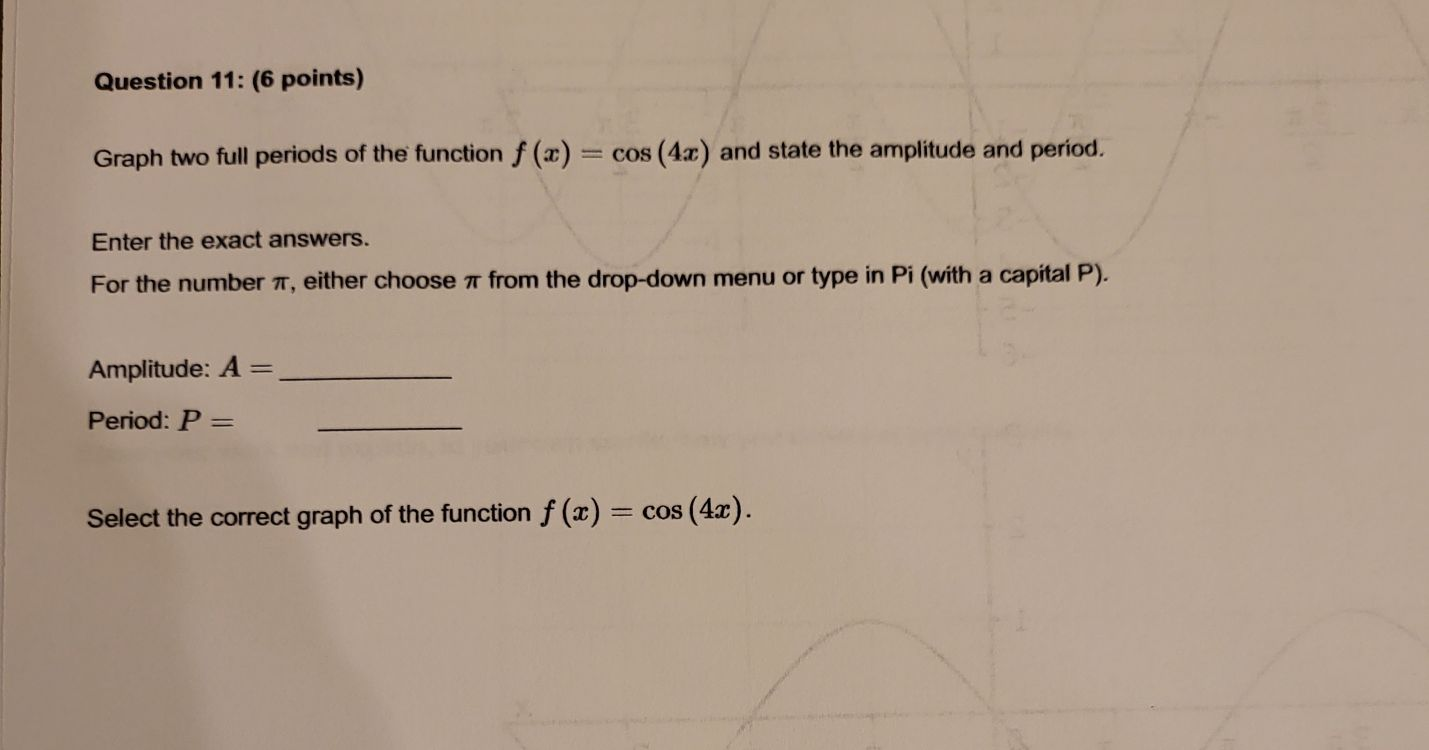### Still have math questions?

Algebra
QuestionGraph two full periods of the function $$f ( x ) = \cos ( 4 x )$$ and state the amplitude and period. Enter the exact answers.

For the number $$\pi$$ , either choose $$\pi$$ from the drop-down menu or type in Pi (with a capital P). Amplitude: $$A =$$

Period: $$P =$$

Select the correct graph of the function $$f ( x ) = \cos ( 4 x )$$ .

$$\frac{\pi }{2}$$